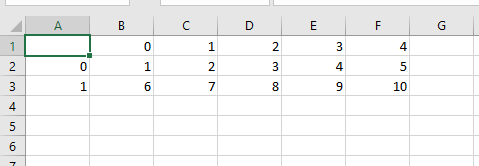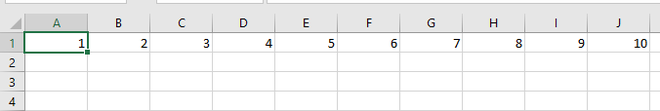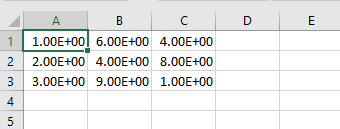# Convert a NumPy array into a csv file

• Last Updated : 02 Sep, 2020

In this article, we are going to see different methods to save an NumPy array into a CSV file. CSV file format is the easiest and useful format for storing data

There are different methods by which we can save the NumPy array into a CSV file

Attention geek! Strengthen your foundations with the Python Programming Foundation Course and learn the basics.

To begin with, your interview preparations Enhance your Data Structures concepts with the Python DS Course. And to begin with your Machine Learning Journey, join the Machine Learning - Basic Level Course

Method 1: Using Dataframe.to_csv().

This method is used to write a Dataframe into a CSV file.

Example: Converting the array into pandas Dataframe and then saving it to CSV format.

## Python3

 `# import necessary libraries``import` `pandas as pd``import` `numpy as np`` ` `# create a dummy array``arr ``=` `np.arange(``1``,``11``).reshape(``2``,``5``)`` ` `# display the array``print``(arr)`` ` `# convert array into dataframe``DF ``=` `pd.DataFrame(arr)`` ` `# save the dataframe as a csv file``DF.to_csv(``"data1.csv"``)`

Output:

```[[ 1  2  3  4  5]
[ 6  7  8  9 10]]
```Method 2: Using numpy_array.tofile().

This method is used to write an array into the file.

Example: Create an array then save into a CSV file.

## Python3

 `# import the necessary library``import` `numpy as np`` ` `# create a dummy array``arr ``=` `np.arange(``1``,``11``)`` ` `# display the array``print``(arr)`` ` `# use the tofile() method ``# and use ',' as a separator``# as we have to generate a csv file``arr.tofile(``'data2.csv'``, sep ``=` `','``)`

Output:

```[ 1  2  3  4  5  6  7  8  9 10]
```Method 3: Using numpy.savetext().

This method is used to save an array to a text file.

Example: Create an array then save as a CSV file.

## Python3

 `# import numpy library``import` `numpy`` ` `# create an array``a ``=` `numpy.array([[``1``, ``6``, ``4``],``                 ``[``2``, ``4``, ``8``],``                 ``[``3``, ``9``, ``1``]])`` ` `# save array into csv file``numpy.savetxt(``"data3.csv"``, a, ``              ``delimiter ``=` `","``)`

Output:My Personal Notes arrow_drop_up